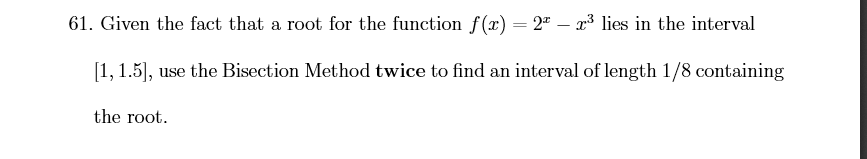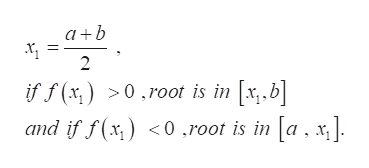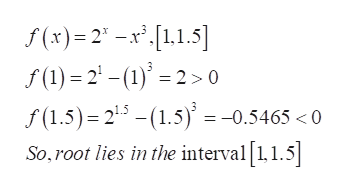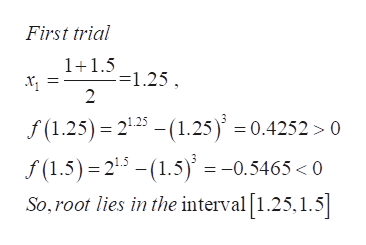# 61. Given the fact that a root for the function f(x) = 2 - 3 lies in the interval1, 1.5,use the Bisection Method twice to find an interval of length 1/8 containingthe root

Questionhelp_outlineImage Transcriptionclose61. Given the fact that a root for the function f(x) = 2 - 3 lies in the interval 1, 1.5, use the Bisection Method twice to find an interval of length 1/8 containing the root fullscreen
check_circle

Step 1

Refer to the question, we have to use the Bisection method and the procedure of bisection method is if f(x) is the function which has root in [a,b] then f(a)>0 and f(b)<0 then it means that the root lies between ‘a’ and ‘b’ .

Then x1=(a+b)/2 , and if  f(x1) >0 ,root is in [x1,b] and if f(x1) <0 ,root is in [a , x1].help_outlineImage Transcriptionclose2 >0.root is in x,.b] and if f(x) 0.root is in [a . x] if f(x,) fullscreen
Step 2

And this process is repeated until we get the required accuracy.

Here provided function is f(x)=2X-X3, in the interval [1,1.5],then follow the above procedure as,help_outlineImage Transcriptionclosef(x)2-x[1.1.5] (1) 2-(1)20 f(1.5) 21.5-(1.5 = -0.5465 <0 So, root lies in the interval 1,1.5| fullscreen
Step 3

Use the first trial of bi...help_outlineImage TranscriptioncloseFirst trial 1+1.51.25 2 f(1.25) 225(1.25) 0.4252> 0 f(1.5) 215-(1.5) = -0.5465 <0 So, root lies in the interval [1.25,1.5] fullscreen

### Want to see the full answer?

See Solution

#### Want to see this answer and more?

Solutions are written by subject experts who are available 24/7. Questions are typically answered within 1 hour.*

See Solution
*Response times may vary by subject and question.
Tagged in

### Calculus